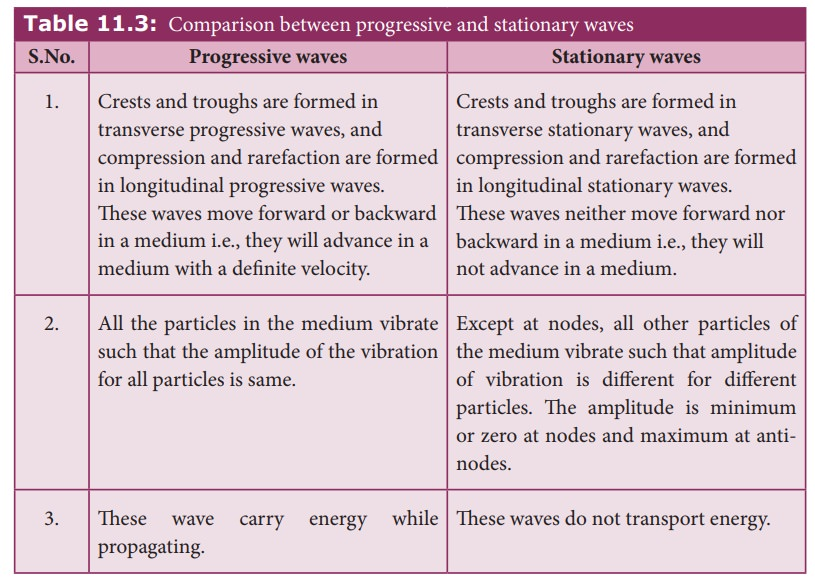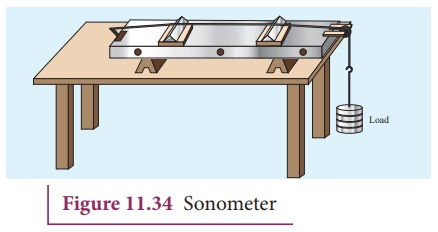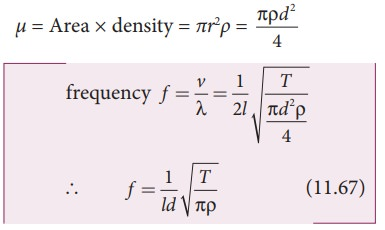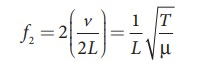Home | | Physics 11th std | Standing Waves or stationary waves

# Standing Waves or stationary waves

When the wave hits the rigid boundary it bounces back to the original medium and can interfere with the original waves.

STANDING WAVES

## Explanation of stationary waves

When the wave hits the rigid boundary it bounces back to the original medium and can interfere with the original waves. A pattern is formed, which are known as standing waves or stationary waves. Consider two harmonic progressive waves (formed by strings) that have the same amplitude and same velocity but move in opposite directions. Then the displacement of the first wave (incident wave) isand the displacement of the second wave (reflected wave) isboth will interfere with each other by the principle of superposition, the net displacement isSubstituting equation (11.59) and equation (11.60) in equation (11.61), we getUsing trigonometric identity, we rewrite equation (11.62) asThis represents a stationary wave or standing wave, which means that this wave does not move either forward or backward, whereas progressive or travelling waves will move forward or backward. Further, the displacement of the particle in equation (11.63) can be written in more compact form,

y(x,t) =cos(ωt)

where, Aʹ = 2Asin(kx), implying that the particular element of the string executes simple harmonic motion with amplitude equals to . The maximum of this amplitude occurs at positions for which

sin(kx) =1where m takes half integer or half integral values. The position of maximum amplitude is known as antinode. Expressing wave number in terms of wavelength, we can represent the anti-nodal positions asand so on.

The distance between two successive anti-nodes can be computed bySimilarly, the minimum of the amplitude A' also occurs at some points in the space, and these points can be determined by setting

sin(kx)= 0 k x = 0,π,2π,3π,… = n π

where n takes integer or integral values. Note that the elements at these points do not vibrate (not move), and the points are called nodes. The nthnodal positions is given by,For n = 0 we have minimum at

x0 = 0

For n = 1 we have minimum at

x1 = λ /2

For n = 2 we have maximum at

x2 = λ

and so on.

The distance between any two successive nodes can be calculated as### EXAMPLE 11.20

Compute the distance between anti-node and neighbouring node.

### Solution

For nth mode, the distance between anti-node and neighbouring node is## Characteristics of stationary waves

(1) Stationary waves are characterised by the confinement of a wave disturbance between two rigid boundaries. This means, the wave does not move forward or backward in a medium (does not advance), it remains steady at its place. Therefore, they are called “stationary waves or standing waves”.

(2) Certain points in the region in which the wave exists have maximum amplitude, called as anti-nodes and at certain points the amplitude is minimum or zero, called as nodes.

(3) The distance between two consecutive nodes (or) anti-nodes is λ/2 .

(4) The distance between a node and its neighbouring anti-node is λ/4.

(5) The transfer of energy along the standing wave is zero.## Stationary waves in sonometer

Sono means sound related, and sonometer implies sound-related measurements. It is a device for demonstrating the relationship between the frequency of the sound produced in the transverse standing wave in a string, and the tension, length and mass per unit length of the string. Therefore, using this device, we can determine the following quantities:

(a) the frequency of the tuning fork or frequency of alternating current

(b) the tension in the string

(c) the unknown hanging mass### Construction:

The sonometer is made up of a hollow box which is one meter long with a uniform metallic thin string attached to it. One end of the string is connected to a hook and the other end is connected to a weight hanger through a pulley as shown in Figure 11.34. Since only one string is used, it is also known as monochord. The weights are added to the free end of the wire to increase the tension of the wire. Two adjustable wooden knives are put over the board, and their positions are adjusted to change the vibrating length of the stretched wire.

### Working :

A transverse stationary or standing wave is produced and hence, at the knife edges P and Q, nodes are formed. In between the knife edges, anti-nodes are formed.

If the length of the vibrating element is l thenLet f be the frequency of the vibrating element, T the tension of in the string and μ the mass per unit length of the string. Then using equation (11.13), we getLet ρ be the density of the material of the string and d be the diameter of the string. Then the mass per unit length μ,### EXAMPLE 11.21

Let f be the fundamental frequency of the string. If the string is divided into three segments l1, l2 and l3 such that the fundamental frequencies of each segments be f1, f2 and f3, respectively. Show that### Solution

For a fixed tension T and mass density µ, frequency is inversely proportional to the string length i.e.## Fundamental frequency and overtones

Let us now keep the rigid boundaries at x = 0 and x = L and produce a standing waves by wiggling the string (as in plucking strings in a guitar). Standing waves with a specific wavelength are produced. Since, the amplitude must vanish at the boundaries, therefore, the displacement at the boundary must satisfy the following conditions

y(x = 0, t) = 0 and y(x = L, t) = 0      (11.68)

Since the nodes formed are at a distance λn/2 apart, we have n(λn/2) = L, where n is an integer, L is the length between the two boundaries and λn is the specific wavelength that satisfy the specified boundary conditions. Hence,Therefore, not all wavelengths are allowed. The (allowed) wavelengths should fit with the specified boundary conditions, i.e., for n = 1, the first mode of vibration has specific wavelength λl = 2L. Similarly for n = 2, the second mode of vibration has specific wavelengthFor n = 3, the third mode of vibration has specific wavelengthand so on.

The frequency of each mode of vibration (called natural frequency) can be calculated.The lowest natural frequency is called the fundamental frequency.The second natural frequency is called the first over tone.The third natural frequency is called the second over tone.Therefore, the nth natural frequency can be computed as integral (or integer ) multiple of fundamental frequency, i.e.,

fn = nf1,      where n is an integer (11.72)

If natural frequencies are written as integral multiple of fundamental frequencies, then the frequencies are called harmonics. Thus, the  first harmonic is f1 = f1 (the fundamental frequency is called first harmonic), the second harmonic is f2 = 2f1, the third harmonic is f3 = 3f1 etc.

### EXAMPLE 11.22

Consider a string in a guitar whose length is 80 cm and a mass of 0.32 g with tension 80 N is plucked. Compute the first four lowest frequencies produced when it is plucked.

### Solution

The velocity of the waveThe length of the string, L = 80 cm=0.8 m The mass of the string, m = 0.32 g = 0.32 × 10-3kg

Therefore, the linear mass density,The tension in the string, T = 80 NThe wavelength corresponding to the fundamental frequency f1 is λ1 = 2L = 2 × 0.8 = 1.6 m

The fundamental frequency f1 corresponding to the wavelength λ1Similarly, the frequency corresponding to the second harmonics, third harmonics and fourth harmonics are

f2 = 2f1 = 559 Hz

f3 = 3f1 = 838.5 Hz

f4 = 4f1 = 1118 Hz

## Laws of transverse vibrations in stretched strings

There are three laws of transverse vibrations of stretched strings which are given as follows:

### (i)   The law of length :

For a given wire with tension T (which is fixed) and mass per unit length μ (fixed) the frequency varies inversely with the vibrating length. Therefore,l×f = C, where C is a constant

### (ii)   The law of tension:For a given vibrating length l (fixed) and mass per unit length μ (fixed) the frequency varies directly with the square root of the tension T,### (iii) The law of mass:

For a given vibrating length l (fixed) and tension T (fixed) the frequency varies inversely with the square root of the mass per unit length μ,Study Material, Lecturing Notes, Assignment, Reference, Wiki description explanation, brief detail
11th Physics : UNIT 11 : Waves : Standing Waves or stationary waves |